CBSE Class 7CBSE
Share

NCERT solutions for Class 7 Mathematics chapter 5 - Lines and Angles

Mathematics for Class 7

NCERT Mathematics Class 7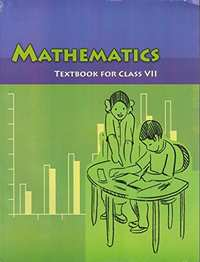Chapter 5: Lines and Angles

Ex. 5.10OthersEx. 5.20

Chapter 5: Lines and Angles Exercise 5.10 solutions [Pages 101 - 103]

Ex. 5.10 | Q 1.1 | Page 101

Find the complement of the following angles: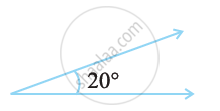Ex. 5.10 | Q 1.2 | Page 101

Find the complement of the following angles: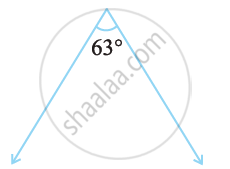Ex. 5.10 | Q 1.3 | Page 101

Find the complement of the following angles: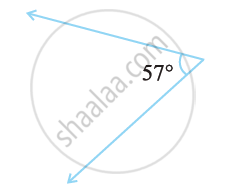Ex. 5.10 | Q 2.1 | Page 102

Find the supplement of the following angles: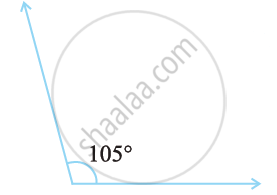Ex. 5.10 | Q 2.2 | Page 102

Find the supplement of the following angles: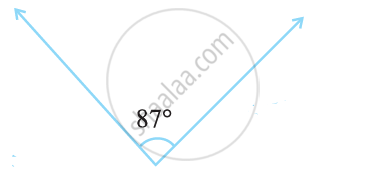Ex. 5.10 | Q 2.3 | Page 102

Find the supplement of the following angles: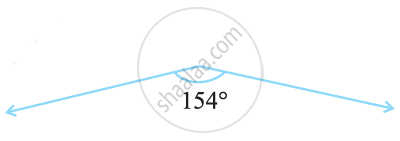Ex. 5.10 | Q 3.1 | Page 102

Identify the given pairs of angles are complementary or supplementary.

65°, 115°

Q 3.2 | Page 102

Identify the given pairs of angles are complementary or supplementary.

63°, 27°

Ex. 5.10 | Q 3.3 | Page 102

Identify the given pairs of angles are complementary or supplementary.
112°, 68°

Ex. 5.10 | Q 3.4 | Page 102

Identify the given pairs of angles are complementary or supplementary.

130°, 50°

Ex. 5.10 | Q 3.5 | Page 102

Identify the given pairs of angles are complementary or supplementary.

45°, 45°

Ex. 5.10 | Q 3.6 | Page 102

Identify the given pairs of angles are complementary or supplementary.

80°, 10°

Ex. 5.10 | Q 4 | Page 102

Find the angle which is equal to its complement.

Ex. 5.10 | Q 5 | Page 102

Find the angle which is equal to its supplement.

Ex. 5.10 | Q 6 | Page 102

In the given figure, ∠1 and ∠2 are supplementary angles. If ∠1 is decreased, what changes should take place in ∠2 so that both the angles still remain supplementary.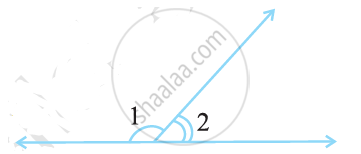Ex. 5.10 | Q 7.1 | Page 102

Can two angles be supplementary if both of them are:acute?

Ex. 5.10 | Q 7.2 | Page 102

Can two angles be supplementary if both of them are:Obtuse?

Ex. 5.10 | Q 7.3 | Page 102

Can two angles be supplementary if both of them are:Right?

Ex. 5.10 | Q 8 | Page 102

An angle is greater than 45°. Is its complementary angle greater than 45° or equal to 45° or less than 45°?

Ex. 5.10 | Q 9 | Page 102

In the adjoining figure:

(1) Is ∠1 adjacent to ∠2?

(2) Is ∠AOC adjacent to ∠AOE?

(3) Do ∠COE and ∠EOD form a linear pair?

(4) Are ∠BOD and ∠DOA supplementary?

(5) Is ∠1 vertically opposite to ∠4?

(6) What is the vertically opposite angle of ∠5?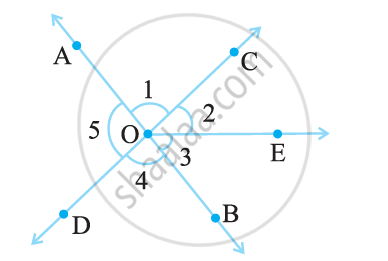Ex. 5.10 | Q 10.1 | Page 102

Indicate which pairs of angles are Vertically opposite angles.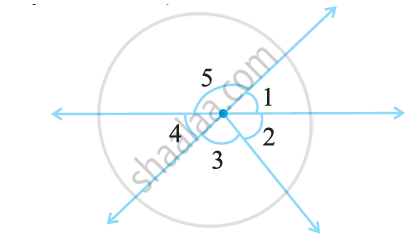Ex. 5.10 | Q 10.2 | Page 102

Indicate which pairs of angles are: Linear pairs.Ex. 5.10 | Q 11 | Page 103

In the following figure, is ∠1 adjacent to ∠2? Give reasons.Ex. 5.10 | Q 12.1 | Page 103

Find the value of the angles xy, and z in the following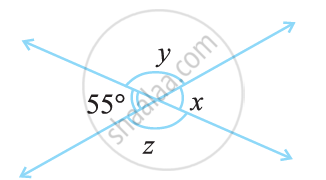Ex. 5.10 | Q 12.2 | Page 103

Find the value of the angles xy, and z in the following: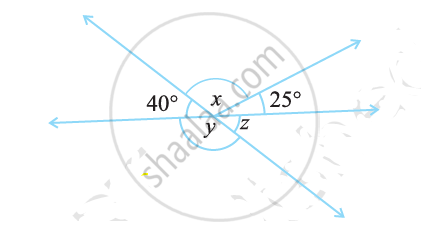Ex. 5.10 | Q 13.1 | Page 103

Fill in the blanks:

If two angles are complementary, then the sum of their measures is _______.

Ex. 5.10 | Q 13.2 | Page 103

Fill in the blanks:

If two angles are supplementary, then the sum of their measures is _______.

Ex. 5.10 | Q 13.2 | Page 103

Fill in the blanks:

If two angles are supplementary, then the sum of their measures is _______.

Ex. 5.10 | Q 13.3 | Page 103

Fill in the blanks:

Two angles forming a linear pair are ______

Ex. 5.10 | Q 13.4 | Page 103

Fill in the blanks:

If two adjacent angles are supplementary, they form a _______.

Ex. 5.10 | Q 13.5 | Page 103

Fill in the blanks:

If two lines intersect at a point, then the vertically opposite angles are always ______

Ex. 5.10 | Q 13.6 | Page 103

Fill in the blanks:

If two lines intersect at a point, and if one pair of vertically opposite angles are acute angles, then the other pair of vertically opposite angles are _______.

Ex. 5.10 | Q 14 | Page 103

In the adjoining figure, name the following pairs of angles.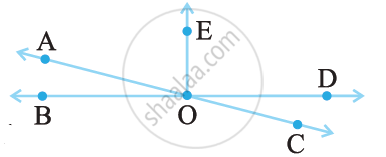1) Obtuse vertically opposite angles

2) Adjacent complementary angles

3) Equal supplementary angles

4) Unequal supplementary angles

5) Adjacent angles that do not form a linear pair

Chapter 5: Lines and Angles Exercise 5.20 solutions [Pages 110 - 111]

Ex. 5.20 | Q 1 | Page 110

State the property that is used in each of the following statements?

1) If a||b, then ∠1 = ∠5

2) If ∠4 = ∠6, then a||b

3)  If ∠4 + ∠5 = 180°, then a||b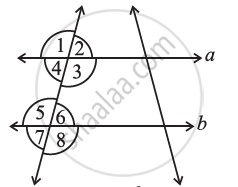Ex. 5.20 | Q 2 | Page 110

In the adjoining figure, identify

(1) The pairs of corresponding angles

(2) The pairs of alternate interior angles

(3) The pairs of interior angles on the same side of the transversal

(4) The vertically opposite angles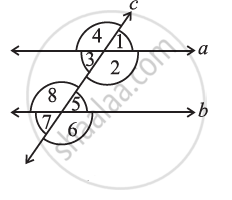Ex. 5.20 | Q 3 | Page 110

In the adjoining figure, || q. Find the unknown angles.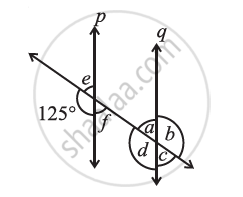Ex. 5.20 | Q 4.1 | Page 111

Find the value of x in the following figures if l || m.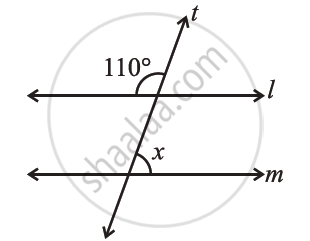Ex. 5.20 | Q 4.2 | Page 111

Find the value of x in the following figure if || m.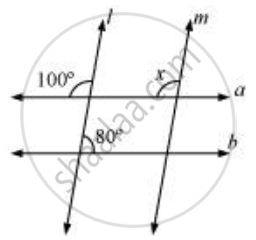Ex. 5.20 | Q 5 | Page 111

In the given figure, the arms of two angles are parallel.

If ∠ABC = 70°, then find

(1) ∠DGC

(2) ∠DEF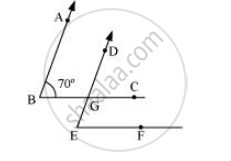Ex. 5.20 | Q 6.1 | Page 111

In the given figure below, decide whether l is parallel to m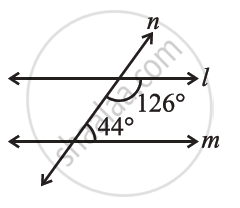Ex. 5.20 | Q 6.2 | Page 111

In the given figure below, decide whether l is parallel to m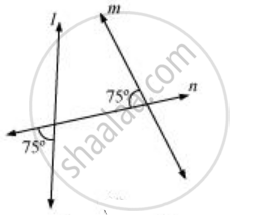Ex. 5.20 | Q 6.3 | Page 111

In the given figure below, decide whether l is parallel to m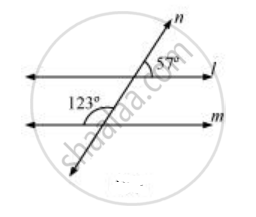Ex. 5.20 | Q 6.4 | Page 111

In the given figure below, decide whether l is parallel to m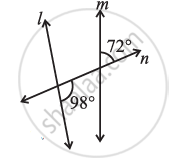Chapter 5: Lines and Angles

Ex. 5.10OthersEx. 5.20

NCERT Mathematics Class 7NCERT solutions for Class 7 Mathematics chapter 5 - Lines and Angles

NCERT solutions for Class 7 Maths chapter 5 (Lines and Angles) include all questions with solution and detail explanation. This will clear students doubts about any question and improve application skills while preparing for board exams. The detailed, step-by-step solutions will help you understand the concepts better and clear your confusions, if any. Shaalaa.com has the CBSE Mathematics for Class 7 solutions in a manner that help students grasp basic concepts better and faster.

Further, we at Shaalaa.com are providing such solutions so that students can prepare for written exams. NCERT textbook solutions can be a core help for self-study and acts as a perfect self-help guidance for students.

Concepts covered in Class 7 Mathematics chapter 5 Lines and Angles are Introduction to Lines and Angles, Complementary Angles, Supplementary Angles, Adjacent Angles, Concept of Linear Pair, Concept of Vertically Opposite Angles, Pairs of Lines - Intersecting Lines, Pairs of Lines - Transversal, Pairs of Lines - Angles Made by a Transversal, Pairs of Lines - Transversal of Parallel Lines, Checking for Parallel Lines.

Using NCERT Class 7 solutions Lines and Angles exercise by students are an easy way to prepare for the exams, as they involve solutions arranged chapter-wise also page wise. The questions involved in NCERT Solutions are important questions that can be asked in the final exam. Maximum students of CBSE Class 7 prefer NCERT Textbook Solutions to score more in exam.

Get the free view of chapter 5 Lines and Angles Class 7 extra questions for Maths and can use Shaalaa.com to keep it handy for your exam preparation

S